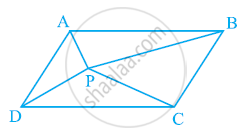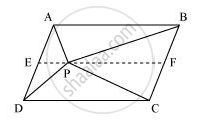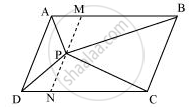# In the given figure, P is a point in the interior of a parallelogram ABCD. Show that (i) ar (APB) + ar (PCD) = 1/2ar (ABCD) (ii) ar (APD) + ar (PBC) = ar (APB) + ar (PCD) - Mathematics

In the given figure, P is a point in the interior of a parallelogram ABCD. Show that

(i) ar (APB) + ar (PCD) = 1/2ar (ABCD)

(ii) ar (APD) + ar (PBC) = ar (APB) + ar (PCD)

[Hint: Through. P, draw a line parallel to AB]#### Solution(i) Let us draw a line segment EF, passing through point P and parallel to line segment AB.

In parallelogram ABCD,

AB || EF (By construction) ... (1)

ABCD is a parallelogram.

∴ AD || BC (Opposite sides of a parallelogram)

⇒ AE || BF ... (2)

From equations (1) and (2), we obtain

AB || EF and AE || BF

Therefore, quadrilateral ABFE is a parallelogram.

It can be observed that ΔAPB and parallelogram ABFE are lying on the same base AB and between the same parallel lines AB and EF.

∴ Area (ΔAPB) = 1/2Area (ABFE) ... (3)

Similarly, for ΔPCD and parallelogram EFCD,

Area (ΔPCD) = 1/2Area (EFCD) ... (4)

Adding equations (3) and (4), we obtain

Area (ΔAPB) + Area (ΔPCD) = 1/2[Area (ABFE) + Area (EFCD)]

Area (ΔAPB) + Area (ΔPCD) = 1/2Area (ABCD)          ........(5)

(ii)Let us draw a line segment MN, passing through point P and parallel to line segment AD.

In parallelogram ABCD,

MN || AD (By construction)                  ... (6)

ABCD is a parallelogram.

∴ AB || DC (Opposite sides of a parallelogram)

⇒ AM || DN                  ... (7)

From equations (6) and (7), we obtain

MN || AD and AM || DN

Therefore, quadrilateral AMND is a parallelogram.

It can be observed that ΔAPD and parallelogram AMND are lying on the same base AD and between the same parallel lines AD and MN.

∴ Area (ΔAPD) = 1/2Area (AMND)                      ... (8)

Similarly, for ΔPCB and parallelogram MNCB,

Area (ΔPCB) = 1/2Area (MNCB)                    ... (9)

Adding equations (8) and (9), we obtain

Area (ΔAPD) + Area (ΔPCB) = 1/2[Area (AMND) + Area (MNCB)]

Area (ΔAPD) + Area (ΔPCB) = 1/2Area (ABCD)        ...........(10)

On comparing equations (5) and (10), we obtain

Area (ΔAPD) + Area (ΔPBC) = Area (ΔAPB) + Area (ΔPCD)

Concept: Theorem: Parallelograms on the Same Base and Between the Same Parallels.
Is there an error in this question or solution?
Chapter 9: Areas of Parallelograms and Triangles - Exercise 9.2 [Page 159]

#### APPEARS IN

NCERT Class 9 Maths
Chapter 9 Areas of Parallelograms and Triangles
Exercise 9.2 | Q 4 | Page 159

Share